HOME plateWON | World!OfNumbersThe Nine Digits Page 2 with some Ten Digits (pandigital) exceptions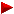Page 1Page 3Page 4Page 5Page 6Page 7

When I use the term ninedigital in these articles I always refer to a strictly zeroless pandigital (digits from 1 to 9 each appearing just once).

Second Page

[ March 1, 2000 ]
Aran Kuntze is studying the occurrences of the nine digits numbers
in the decimal expansion ofSo for instance (taken three at random from her list of 41)

The string 165429837 was found at position 10552019
The string 654321987 was found at position 14597746
The string 976543182 was found at position 22314906

Puzzle 1
Aran (from Finland) is also hoping to find a palindromic position but
had no luck so far. Can you give her a helping hand in her search ?

Puzzle 2
I propose Aran or any other puzzler to try to find a solution that occurs
at a nine digits position. Beware that it will be a hard job as one has to have
access to the first 1.000.000.000 digits ofto scan through.
The string some nine digits number was found at position some nine digits number ?

[ February 14, 2000 ]
Once again G. L. Honaker, Jr. made an interesting discovery

This time it is about 6 primes in Arithmetic Progression
with a common difference of... our  pandigital  number !

5077
9876548287
19753091497
29629634707
39506177917
49382721127
The common difference is 9876543210.

See Sloane's A058908.

Who can construct such sequences with 7 or more primes in AP ?

From Felice Russo's sequence A039667

There exist only four numbers n so that
juxtaposition of  n, 2n and 3n forms a  nine digits number.

192 384 576 : 192384576
219 438 657 : 219438657
273 546 819 : 273546819
327 654 981 : 327654981

[ September 16, 1999 ]
Two new G. L. Honaker, Jr. discoveries !

The sum of the first 10701 consecutive prime numbers is a nine digit anagram.
572469138
in Honaker's words pandigital (excluding the zero)
or in Weisstein's words zeroless pandigital.

The sum of the first 32423 consecutive prime numbers is pandigital.
5897230146
in this case pandigital means including all 10 digits exactly once.
Note that 32423 is the only palindromic prime that produces such a number.
Featured in Prime Curios! 5897230146

Further information
in Sloane's database : A049442, A049443 and A049446.
in Weisstein's Math Encyclopedia Pandigital Number.

[ November 11, 2022 ]
Alexandru Petrescu explores other paths to reach more nine- and pandigital results. Here is his contribution.
He discovered that the sum of the first 59295 (palindromic!) consecutive composites is a ninedigit anagram !

n sum   n sum   n sum The sum of the first n consecutive primenumbers is a ninedigit anagram The sum of the first n consecutive compositenumbers is a ninedigit anagram The sum of the first n consecutive primenumbers is a pandigital anagram The sum of the first n consecutive compositenumbers is a pandigital anagram 8971 394521678 14829 125376498 14353 1063254978 47554 1267389504 10474 547128369 14847 125678493 18572 1829360475 49237 1358079462 10701 572469138 16895 162394758 22876 2835410967 49604 1378269450 [#3] 21146 253486197 25212 3478029561 53356 1593207684 23692 317654892 26799 3954061782 56449 1782046395 25867 378159264 27803 4271593608 56645 1794365802 28643 462983715 28752 4583260179 57328 1837624590 29389 487231695 30510 5190863247 57576 1853460927 30418 521694738 32011 5741092638 59295 1965072348 33681 638719254 32423 5897230146 62248 2164385079 34219 659143728 32515 5932401786 65250 2376809514 41292 957361482 35137 6980571324 66989 2504396871 41417 963127458 37055 7803615924 67828 2567140389 41454 964837512 [#13] 68124 2589460713 [#14] 69621 2703816459 75626 3187265940 79023 3478256901 80736 3629784051 83062 3840691257 83875 3915807462 87976 4305791628 88174 4325086179 90241 4529068317 92224 4729156380 92294 4736298501 95443 5063128749 96581 5183906247 101059 5672914308 104992 6120485379 105438 6172308459 105974 6234875019 119684 7942058361 122491 8316924705 125974 8794052136 127167 8960524713 128445 9140586723 130042 9368104574 131084 9518046237 132572 9734215086 132614 9740351862 132648 9745321086 [#41]

An extraordinary find !

9135728461371731 An extraordinary find by Carlos Rivera [ Visit 'PP&P' Puzzle 41 ]He succeeded in amalgamating four concepts :the Number of the Beast (666) as the quotient,the nine_digits (the numerators)the palindromes (the denominators)the primes - e.g. 1371731 versus the composites - e.g. 396693

[ December 12,1998 ]
Add up the values (A = 1, B = 2, C = 3, etc.) of the letters
of the written out numbers (in English)

From Carlos Rivera's Puzzle 33 of his PP&P website

A result from Carlos Rivera's work on adding the letters of written_out numbers
(in English) is that he proved that these two 9-digit numbers are equal !
The palindromic construction of the equation is a free bonus.

123456789 = 987654321

ONE HUNDRED TWENTY THREE MILLION FOUR HUNDRED FIFTY SIX THOUSAND SEVEN HUNDRED EIGHTY NINE
O+N+E+H+U+N+D+R+E+D+T+W+E+N+T+Y+T+H+R+E+E+M+I+L+L+I+O+N+F+O+U+R+H+U+N+D+R+E+D+F+I+F+T+Y+S+I+X+
T+H+O+U+S+A+N+D+S+E+V+E+N+H+U+N+D+R+E+D+E+I+G+H+T+Y+N+I+N+E
= 964

And this value 964 is also the total of the second nine digits number !

NINE HUNDRED EIGHTY SEVEN MILLION SIX HUNDRED FIFTY FOUR THOUSAND THREE HUNDRED TWENTY ONE
N+I+N+E+H+U+N+D+R+E+D+E+I+G+H+T+Y+S+E+V+E+N+M+I+L+L+I+O+N+S+I+X+H+U+N+D+R+E+D+F+I+F+T+Y+F+O+U+R+
T+H+O+U+S+A+N+D+T+H+R+E+E+H+U+N+D+R+E+D+T+W+E+N+T+Y+O+N+E
= 964
[ Quod Erat Demonstrandum  ;-) ]

The same method can be applied to prove that :
964 = 469273 = 372etc. but I leave that as an exercice for the curious puzzlers amongst you !

What is the 10-digits number
[from alt.math.recreational]

Source : “Mathematical Circus”, Martin Gardner, Penguin Books, 1979, p.128 & 135(solution)

Self-Descriptive Number
Autobiographical numbers : A046043

A pandigital problem
0123456789

In the 10 cells of the above figure inscribe a 10-digit number such that the digit in the first cell indicates the total number
of zeros in the entire number, the digit in the cell marked '1' indicates the total of 1's in the number, and so on to the last cell,
whose digit indicates the total number of 9's in the number. (zero is a digit, of course). The answer is unique.

How to arrive at this unique solution ?Number Problem #1 by QYVProblem 4 by John Scholes

[ November 10, 1998 ]
Puzzle : Juxtaposition of prime factors is a Nine Digits number

The smallest number whereby the juxtaposition (or concatenation if you like)
of its prime factors is a nine digits number is :
2992890 = 2 x 3 x 5 x 67 x 1489 or juxtaposed 235671489.
And here is the largest one :
842696243 = 8641 x 97523 or juxtaposed 864197523.

The smallest pandigital number :
15618090 = 2 x 3 x 5 x 487 x 1069 or juxtaposed 2354871069.

The puzzle questions are now :
[A] Find the largest number whereby the juxtaposition of its prime factors
is a pandigital number (the zero allowed).
[B] What are the smallest and largest juxtaposed nine digits or pandigital numbers ?
[C] What are the smallest and largest numbers for each possible 'n' prime factors whose
juxtaposition is a nine digits or pandigital numbers ?
[D] Are there nine digits or pandigital numbers whose prime factors when juxtaposed yield
another nine digits or pandigital number ?

[ October 4, 1998 ]
Puzzle : Root Extracting Nine Digits numbers

The smallest 9-digits number so that the decimal part of its square root also starts with a 9-digits number is :

`135649728^(1/2) = 11646,876319425 736908...`
Here are more random solutions. E.g.:
```
235916748^(1/2) =  15359,581634927 430535...
317625984^(1/3) =    682,294715863 639889...
413786925^(1/4) =    142,624518379 632388...
529638741^(1/5) =     55,564312978 124193...
613824957^(1/6) =     29,152384967 733170...
713625894^(1/7) =     18,398461752 548250...
893257614^(1/8) =     13,148375269 564618...
926374815^(1/9) =      9,915386274 757628...
```
The puzzle question is now :
Find a root of a 9-digits number so that
[A] the same 9-digits number reappears (if none exists then the one with most digit-matches)
or so that
[B] the same 9-digits number but reversed appears
immediately after the decimal point (the 'comma' for us Europeans) of this root.
Note that you may choose roots other than the square root (of the form '1/n').

[ Don't ask for solutions via e-mail as this is an open puzzle meaning I haven't a solution myself. ]
Happy hunting !

If you want to know the integers such that in the decimal representations of the square root of those integers,
the digits to the immediate right of the decimal point are 123456789 and 987654321 then visit the archives of
the Southwest Missouri State University's Problem Corner - Solution to Problem #14 !

Maybe at this point you would like to know the answer to the following
question posed by Carlos Rivera

Let`s suppose that N is a 9 digits number: what is the minimal
arithmetical test (if it exists) that we need to apply to N for
testing if N is an anagram of the 9-digits number 123456789 ?

Carlos explains his best solution for that problem (in UBASIC)

Let's suppose you have a number N of 9 digits, N = d1d2d3d4d5d6d7d9 :
If prm(d1) * prm(d2) * prm(d3) *... * prm(d8) * prm(d9) =
2 * 3 * 5 * 7 * 11 * 13 * 17 * 19 * 23 = 223092870
then N is an anagram;
If the product differs from 223092870
then N is not an anagram.

Other smaller productnumbers can easily be calculated for testing anagrams of less than 9 digits numbers. Beautiful!
Carlos, your best solution is most elegant. That way, you also found an original application for the uniqueness of primes.

Extracting the Nine Digits from the Number of the Beast 666

This goes as follows :

```  Sum_Of_Digits{6^6} = 27      Number_Of_Digits = 5
Sum_Of_Digits{66^66} = 531    Number_Of_Digits = 121
Sum_Of_Digits{666^666} = 8649  Number_Of_Digits = 1881 [P. De Geest]```

The combination of all three numbers miraculously shows all the nine digits in a row 275318649 !
Note also that each time the numbers of digits of the next result appears to be a palindromic number.
Alas, the pattern stops with the third one because

`Number_Of_Digits{6666^6666} = 25490`
and 25490 is not palindromic. Nor are the following terms 321591, 3882602, 45492721, etc.

From Nine Digit Basenumbers via Nine Digit Powers arriving at Palindromes

Here is one of my recent research projects.
It makes a link between palindromes, the 'nine digits' and powers :
Task : Find a construction of the form :

Aa + Bb + Cc + Dd + Ee + Ff + Gg + Hh + Ii = P

whereby P is a palindromic number.
The letters A to I represent the nine digits (1 to 9) and all the nine digits must be used exactly once.
The order is unimportant.
Idem dito for the exponents a to i.

Some examples (two extremes and a random one) :

11 + 22 + 33 + 44 + 55 + 66 + 77 + 88 + 99 = 405071317

19 + 28 + 37 + 46 + 55 + 64 + 73 + 82 + 91 = 11377

49 + 86 + 67 + 14 + 35 + 23 + 51 + 98 + 72 = 43851251

The following table shows the smallest and the largest of the 223 palindromic combinations I found.
[ In fact the search yielded exactly 211 different palindromes. ]
The third row shows a special and unique palindrome that can be written in exactly two ways
so that all the exponents differ vis-à-vis the basenumbers of the two combinations.

Smallest 18 + 29 + 37 + 46 + 55 + 61 + 74 + 83 + 92 15 + 26 + 32 + 41 + 58 + 64 + 73 + 87 + 99 17 + 28 + 32 + 49 + 56 + 61 + 73 + 85 + 94 18 + 26 + 39 + 41 + 54 + 67 + 75 + 83 + 92

From Palindromes via Fibonacci arriving at Nine Digits

As palindromes are my cup of tea allow me to continue this section with them.
What I try to accomplish here is to establish a relationship between three known mathematical concepts.
Via Fibonacci iteration and starting from a palindromic number arriving at a nine digit number !
For the moment I haven't found a palindrome that transforms into 123456789 or its reversal 987654321.
In total there are 68 palindromes that yield 9-digits numbers
The smallest one is 4004 and the largest one is 437606734 ¬

1
4004
4005
8009
12014
20023
32037
52060
84097
136157
220254
356411
576665
933076
1509741
2442817
3952558
6395375
10347933
16743308
27091241
43834549
70925790
114760339
185686129
300446468
486132597

= smallest
1
630036
630037
1260073
1890110
3150183
5040293
8190476
13230769
21421245
34652014
56073259
90725273
146798532
1
1559551
1559552
3119103
4678655
7797758
12476413
20274171
32750584
53024755
85775339
138800094
224575433
363375527
587950960
951326487
1
4187814
4187815
8375629
12563444
20939073
33502517
54441590
87944107
142385697
1
4870784
4870785
9741569
14612354
24353923
38966277
63320200
102286477
165606677
267893154
1
6097906
6097907
12195813
18293720
30489533
48783253
79272786
128056039
207328825
335384864
542713689
1
6834386
6834387
13668773
20503160
34171933
54675093
88847026
143522119
232369145
375891264
1
9530359
9530360
19060719
28591079
47651798
76242877
123894675
68
in total
1
82466428
82466429

164932857
1
437606734
437606735
875213469

= largest

Inspired ? I hope that the readers will find more of these transformational RECREATIONAL FACTS.
For instance :
Who can find a palindrome that transforms into another palindrome via Fibonacci iteration ?
Or :
Who can find a startnumber that transforms via Fibonacci iteration into some other
operational result of that startnumber ?

[ September 11, 2005 ]
Investigation and a new challenge by Carlos Rivera

For your Fibonacci and Pandigital page, specially to your statement 'For the moment I haven't found
a palindrome that transforms into 123456789 or its reversal 987654321.
' what I can say is that
NO palindrome can touch these numbers. Here are my results with some extra's as well :

 15432098 15432099 30864197 46296296 77160493 123456789 61728394 61728395 123456789 493827160 493827161 987654321 110972395 110972396 221944791 332917187 554861978 887779165 1442641143 2330420308 3773061451 6103481759 9876543210 127932098 127932099 255864197 383796296 639660493 1023456789 511728394 511728395 1023456789The only strange thing is that I was thinking that no two fibonacci series,
starting with two distinct initial numbers, could hit the same number. Was I wrong ?
Perhaps the statement is true for any k term of fibonacci sequence (a0, a1, a2, a3, ...) if k>2, ...

Saludos. Regards.

 [ February 18, 2022 ] Daniel Hardisky (email) would like you to find “Generalized Fibonacci Sequences” and “Generalized Tribonacci Sequences” and find the maximum differences in increasing order. Daniel himself was surprised at the large 'gaps' between successive terms. His collage on a placard hereunder provides more information on the subject. Are you up for his challenge ?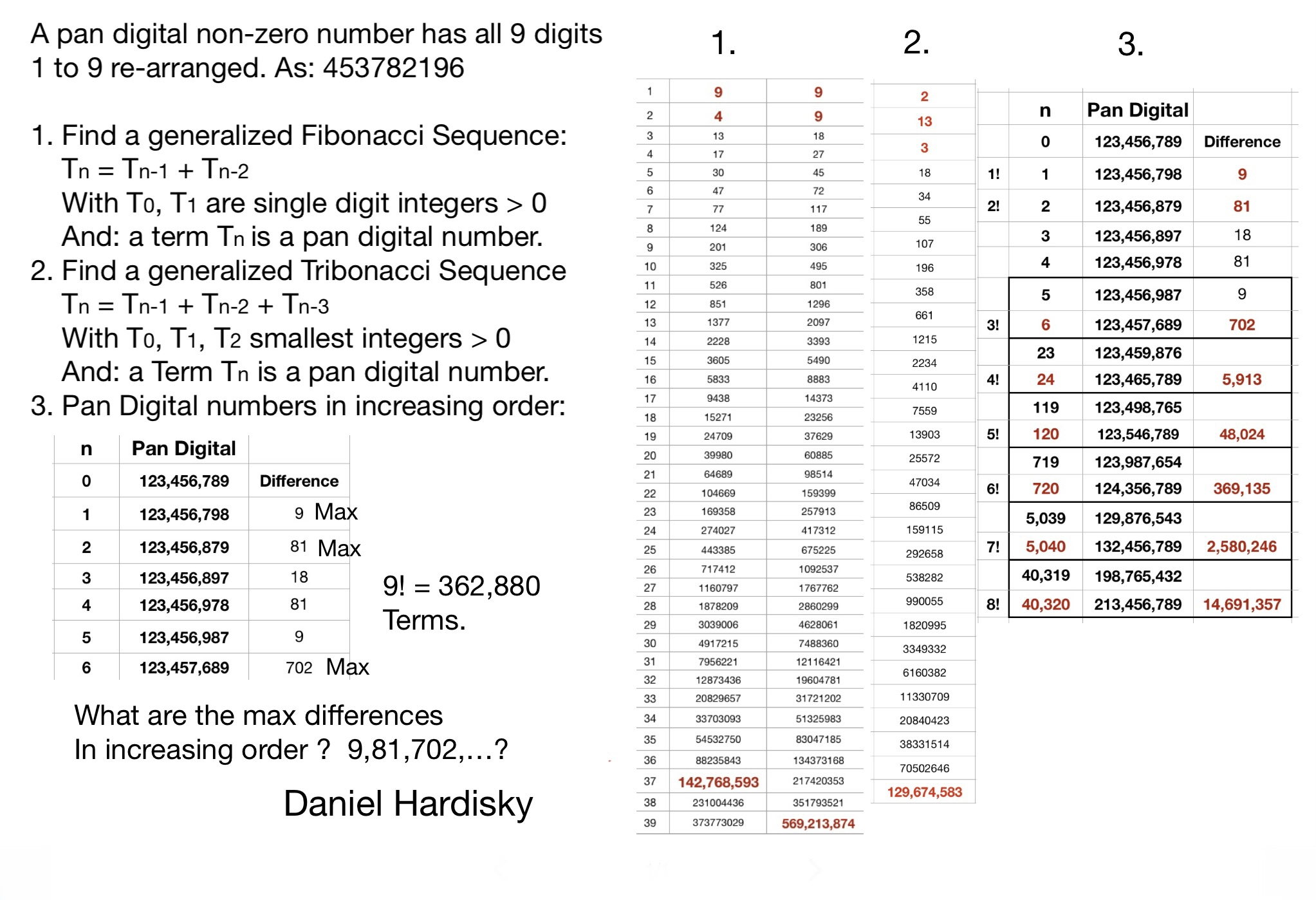Powers of ninedigitals

A small booklet called "Rekenraadsels" from Deltas vrije-tijd-reeks [1981 - ISBN 90-243-2545-5]
inspired me to start this nine digit topic.

The first two numbers multiplied together deliver a result equal to the square of another number
also containing all nine digits
246913578 x 987654312 = 4938271562

Use the digits from 1 to 9 once to form two numbers, so that one number is twice the other one
6729 x 2 = 13458

There are more solutions then the one given above.
Michael Winckler published the following puzzle a while ago (Puzzle No. 121) :

For each ratio 1/2, 1/3, ..., 1/9 find two integers a and b such that
1. a/b has that ratio and that
2. in forming a and b each digit 1 up to 9 is used exactly once.
All possible solutions to this puzzle were sent in by Carlos Rivera shortly after.

0123456789 or pan- and ninedigital diversions

Many more digital combinations can be found in “Madachy's Mathematical Recreations” from
Joseph S. Madachy [Dover N.Y., 1979 - ISBN 0-486-23762-1, pp. 156-162]

I'll give a few excerpt to whet your appetite.
291548736 = 8 x 92 x 531 x 746
124367958 = 627 x 198354 = 9 x 26 x 531487

A square that yields all the nine digits twice !
3351801362 = 112345723568978496

[ May 11, 2008 ]
Investigation by Peter Kogel

Hi Patrick,

In Joseph Madachy's “Mathematical Recreations”  [ on page 159 ] he presents the following result
and asks whether there are more results.

246913578 x 987654312 = 493827156 x 493827156

i.e. A x B = C2 where A, B and C are zero-less pandigital numbers.

Not being one to allow such a challenge to pass by, I set about searching for other
9 and 10 digit pandigital examples.

I was a bit disappointed to eventually discover that there are well over 12000 such solutions
because this somehow seemed to dilute the aesthetic nature of the puzzle. The large number of
solutions though does prove to be a veritable gold mine of supplementary results.

Zero-less solutions# 620
Pandigital solutions# 6619
Mixed solutions# 5587

Total solutions# 12826

Zero-less smallest
2315976842 = 164938572 x 325196748
Zero-less largest
6594187322 = 769321854 x 897542163

Pandigital smallest
13789650422 = 1280467539 x 1485039276
Pandigital largest
83267904512 = 7450286193 x 9306412857

There are 40 examples where the sum of A and B is also zero-less and 404 examples
where the sum is pandigital. E.g. the smallest for each type is :

2469135782 = 123456789 x 493827156
617283945 =  123456789 + 493827156

20539147682 = 1026957384 x 4107829536
5134786920 =  1026957384 + 4107829536

Note that the number C is actually the geometric mean of A and B !
I found that there are 36 solutions where the arithmetic mean is also pandigital. E.g.

Geometric Mean ( 1076539482, 4306157928 ) = 2153078964
Arithmetic Mean ( 1076539482, 4306157928 ) = 2691348705

There are no such solutions for zero-less numbers.

There are 19 examples where both A and B are pandigital square numbers and
one example where they are zero-less squares.
 358532 = 1285437609 234392 = 549386721 714332 = 5102673489 x 272732 = 743816529 x 25610873492 6392518472

To the best of my knowledge these results have never been noted before. I find this surprising
considering that the 30 zero-less squares and 87 pandigital squares are very well known.
BTW the first square on the LHS is a palindrome !

I found two solutions where C2 is also doubly pandigital; i.e. it contains each digit twice: viz.

36729805142 = 1836490257 x 7345961028
36729805142 = 13490785856223704196

However, the second solution of this type is the 'pièce de résistance' for I was
elated to note that A + B is also pandigital.

 1854763209 + 7419052836 = 9273816045 37095264182 = 1854763209 x 7419052836 37095264182 = 13760586245839910724

Regards,
Peter Kogel (alias Peter Pan).

[ January 21, 2009 ]
Reversal investigations by Peter Kogel

Hi P@rick,

The reversal relationship you found for the pan x pan = pal investigation
(see bottom part of the webpage at twopan.htm)
led me to wonder whether I couldn't use the same technique for the
pan x pan = pan^2 project I ran last year (see above).
I was delighted to find 6 solutions; viz:

 4297315862 = 859463172 x 214865793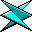397568412 x 1590273648 = 7951368242
 4935827162 = 987165432 x 246791358853197642 x 3412790568 = 17063952842
 13569024782 = 2713804956 x 678451239932154876 x 3728619504 = 18643097522
 13957026842 = 2791405368 x 697851342243158796 x 972635184 = 4863175922
 14590273682 = 2918054736 x 729513684486315927 x 1945263708 = 9726318542
 30975218642 = 6195043728 x 15487609322390678451 x 9562713804 = 47813569022

There are also 23 solutions where the square itself can be reversed; e.g.

 Smallest 261845739 x 1047382956 = 52369147828741963252 = 4370981625 x 174839265
 Largest 175264389 x 4381609725 = 87632194525491236782 = 1098247356 x 274561839

Undoubtedly best of all is the following remarkable result
where each ninedigital number is reversed (unique case):Forward Backward 159723648 846327951 x x 489153672 276351984 = = 2795163842 4836159722

Regards,
Pete Kogel

I've got this from rec.puzzles

There is a 9-digit number in which the digits 1 through 9 appear exactly once.
If you only take the first N digits from the left, the number you're left with is
divisible by that same value N. What is this unique number ?
Visit the Palindromic Puzzle's page for a quick access to the solution.

Ninedigital tile-layers

A tile-layer has exactly 123456789 tiles and has to make a rectangle that best approaches a neat square.
No tiles are fractured or left out. [ Dutch Source : original but dead link = http://www.win.tue.nl/math/dw/ida/h1s4.html#tegelfactor ]

He chooses this rectangle : 11409 x 10821
Can you prove that this is the best solution ?
And what if you gave our man 987654321 tiles to play with ?
[ January 8, 2007 ]
B.S.Rangaswamy (email) got involved in the above puzzle.
He could give the tile-layer a very narrow straight pathway, measuring 2601 x 379721
(length almost 146 times that of the width) to house 987654321 tiles. There can be no
wider path since 379721 happens to be the largest prime factor of 987654321.
Thanks for providing me an opportunity to get involved in this puzzle and express my view.

Also from rec.puzzles

This one from a book by 'L.A. Graham'
Using all the 9 digits from 1 to 9 and only once, what two numbers multiplied
give the largest product ? Such as 12345 times 6789.
87531 x 9642 = 843973902

Source : All the Math that's Fit to Print
by Keith Devlin [ Chapter 17 p.44 ]

Arrange the digits 1 to 9 into two numbers, one of which is the square of the other.

The two possible solutions are
5672 = 321489
and
8542 = 729316

A Selection of Magic Squares Websources

Magic Squares by Harvey Heinz
Magic Squares - the ultimate database by Mutsumi Suzuki
What is a Magic square ? by Allan Adler
Magic squares - Building a 9-Cell Square by Suzanne Alejandre
More than Magic squares by Ivars Peterson
Magic Square by E. Weisstein
Creating magic squares by Zimaths

Construct a Magic Square using all the digits from 1 to 9

816
357
492

Note that all the rows and columns and diagonals add up to 15 (fifteen).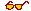Visit WONplate 9 and WONplate 14 to see two beautiful Magic Squares constructed only with palindromic numbers !
Pity that the magic sums are not palindromic as well !

From All the Math that's Fit to Print
by Keith Devlin pages 180 and 182

Find a palindrome that when multiplied with 123456789
gives a number that ends with its reversal ...987654321.
Answer : that number is 989010989
The result is 122100120987654321

From Dictionary of Curious and Interesting Numbers (revised edition 1997)
by David Wells page 149

The minimum sum of ninedigital 3-digit primes, 149 + 263 + 587 = 999 and PALINDROMIC.
Can you find more of these fun facts ?!

MORELAND PI (anagram of PALINDROMES) welcomes the nine digits

Pi search for the nine digits
Consult the 100.000.000 digits of PI and try to locate the nine digits.
Results
The string 123456789 did not occur in the first 100000000 digits of pi after position 0.
The string 987654321 did not occur in the first 100000000 digits of pi after position 0.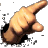This is a subtopic where I need your help. After all, of the 362.880 possible
combinations I only checked the above two...

Martin Gardner wrote a chapter about Random Numbers in his book "Mathematical Carnival".
Here's a small excerpt [ p.167 ]

... It (the brain) acquires its ability to "see" patterns only after years of experience during which
the patterned external world imposes its order on the brain's tabula rasa. It is true, of course,
that one is surprised by a sequence of 123456789 in a series of random digits because such a
sequence is defined by human mathematicians and used in counting, but there is a sense in which
such sequences correspond to the structure of the outside world...
Recently a NEW RECORD is set in the calculation of PI.
Declared record : 51.539.600.000 decimal digits
The Brouwer-Heyting Sequence
Table of current records for the computation of constants

Here are some interesting pandigital sequences :
0123456789 : from 17.387.594.880-th of pi
9876543210 : from 21.981.157.633-th of pi

Curiously this sum is palindromic and repunital

987654321 + 1 + 123456789 = 1111111111

From the book The Penguin Dictionary of Curious and Interesting Numbers
Surprising facts by David Wells

The difference between 123456789 and 987654321 is an anagram of the ninedigital : 864197532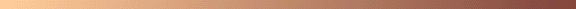The smallest ninedigital square is
118262 = 139854276
The largest ninedigital square is
303842 = 923187456
Albert H. Beiler lists 30 of them (from a total of 83) in his book :
"Recreations in the Theory of Numbers" [ Second Ed. Table 64 p. 148 ].
The squares are composed of the digits 1 up to 9.
11826, 12363, 12543, 14676, 15681, 15963, 18072, 19023, 19377, 19569,
19629, 20316, 22887, 23019, 23178, 23439, 24237, 24276, 24441, 24807,
25059, 25572, 25941, 26409, 26733, 27129, 27273, 29034, 29106, 30384
Here are the 53 numbers left out from the table :
These squares are composed of 9 different digits whereby zero is allowed !
10124, 10128, 10136, 10214, 10278, 12582, 12586, 13147, 13268, 13278,
13343, 13434, 13545, 13698, 14098, 14442, 14743, 14766, 15353, 16549,
16854, 17252, 17529, 17778, 17816, 20089, 20513, 20754, 21397, 21439,
21744, 21801, 21877, 21901, 22175, 22456, 23113, 23682, 23728, 23889,
24009, 25279, 26152, 26351, 27105, 27209, 27984, 28171, 28256, 28346,
28582, 28731, 29208
Note that only one of them is palindromic :
285822 = 816930724The smallest pandigital square is
320432 = 1026753849
The largest pandigital square is
990662 = 9814072356
Albert H. Beiler list 10 of them (from a total of 87) in his book :
"Recreations in the Theory of Numbers" [ Second Ed. Table 65 p. 148 ].
32043, 32286, 33144, 35172, 39147, 45624, 55446, 68763, 83919, 99066
Here are the 77 numbers left out from the table :
35337, 35757, 35853, 37176, 37905, 38772, 39336, 40545, 42744, 43902,
44016, 45567, 46587, 48852, 49314, 49353, 50706, 53976, 54918, 55524,
55581, 55626, 56532, 57321, 58413, 58455, 58554, 59403, 60984, 61575,
61866, 62679, 62961, 63051, 63129, 65634, 65637, 66105, 66276, 67677,
68781, 69513, 71433, 72621, 75759, 76047, 76182, 77346, 78072, 78453,
80361, 80445, 81222, 81945, 84648, 85353, 85743, 85803, 86073, 87639,
88623, 89079, 89145, 89355, 89523, 90144, 90153, 90198, 91248, 91605,
92214, 94695, 95154, 96702, 97779, 98055, 98802
David W. Wilson pointed out to me that three amongst them are palindromic :
358532 = 1285437609
846482 = 7165283904
977792 = 9560732841Our nine digit number 123456789 can be multiplied with 2, 4, 5, 7 and 8
to become a nine digits anagram !
x 2 = 246913578
x 4 = 493827156
x 5 = 617283945
x 7 = 864197523
x 8 = 987654312 note the reversal of the two last digits !

Our nine digit reversal number 987654321 multiplied with 2, 4, 5, 7 and 8
becomes a pandigital number (The nine familiar digits plus zero !).
x 2 = 1975308642
x 4 = 3950617284
x 5 = 4938271605
x 7 = 6913580247
x 8 = 7901234568Find a nine digit number that gives primes
whenever any one digit is dropped

This is a mathproblem once solved by Carlos Rivera

The number 123456789 is a bad example as it produces only primes when the digits 1 and 4 are dropped.
All the others are composite.

123456789 and 123456789   ( 23456789 and 12356789 are prime ! )

The number 987654321 is also a bad candidate as it produces only primes when the digits 2 and 5 are dropped.
All the others are composite.

987654321 and 987654321

Carlos Rivera came to rescue me [ July 8, 1998 ]. He wrote some code and let it ran for three hours.
As a result of this he could tell me that there are no solutions whereby 9, 8 or 7 primes shows up.
The following ninedigit number is prime '6' times when one of its digits is dropped :
126874359 ( 126874359, 126874359, 126874359, 126874359, 126874359, 126874359 )
The table below displays all thirteen solutions.

Ninedigit Number  '6' digits droppings
1268743591,2,8,7,4,5
1496825731,4,8,2,5,7
1827469531,8,2,7,4,5
1925648731,2,5,4,8,7
3824765198,2,4,7,5,1
4128695374,1,2,8,5,7
4289657314,2,8,5,7,1
4538276914,5,8,2,7,1
4572638194,5,7,2,8,1
5643287915,4,2,8,7,1
6371548297,1,5,4,8,2
6945328714,5,2,8,7,1
8642317598,4,2,1,7,5

[ April 17, 2022 ] Thanks, Alexandru Dan Petrescu for spotting an error in the first entry of the table
whereby the digits 2 and 6 were swapped.
When 3, 6 or 9 is dropped it produces always a multiple of 3, because every ninedigit is a multiple of 9!

```

```

Contributions

David W.Wilson (email) - go to topic - [ Fri, April 10, 1998 ].

Carlos Rivera (email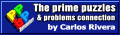from Nuevo León, México.
- go to topic 1 - [ Wed, July 8, 1998 ].
- go to topic 2 - [ Sun, September 11, 2005 ].

Aran Kuntze (email) from Finland - go to topic - [ March 2000 ].

B.S.Rangaswamy (email) from India - go to topic - [ Januari 2007 ].

Peter Kogel (email) from South Africa - go to topic - [ May 11, 2008 ].

Peter Kogel (email) from South Africa - go to topic - [ January 21, 2009 ].

```

```

[TOP OF PAGE]

Patrick De Geest - Belgium- Short Bio - Some Pictures